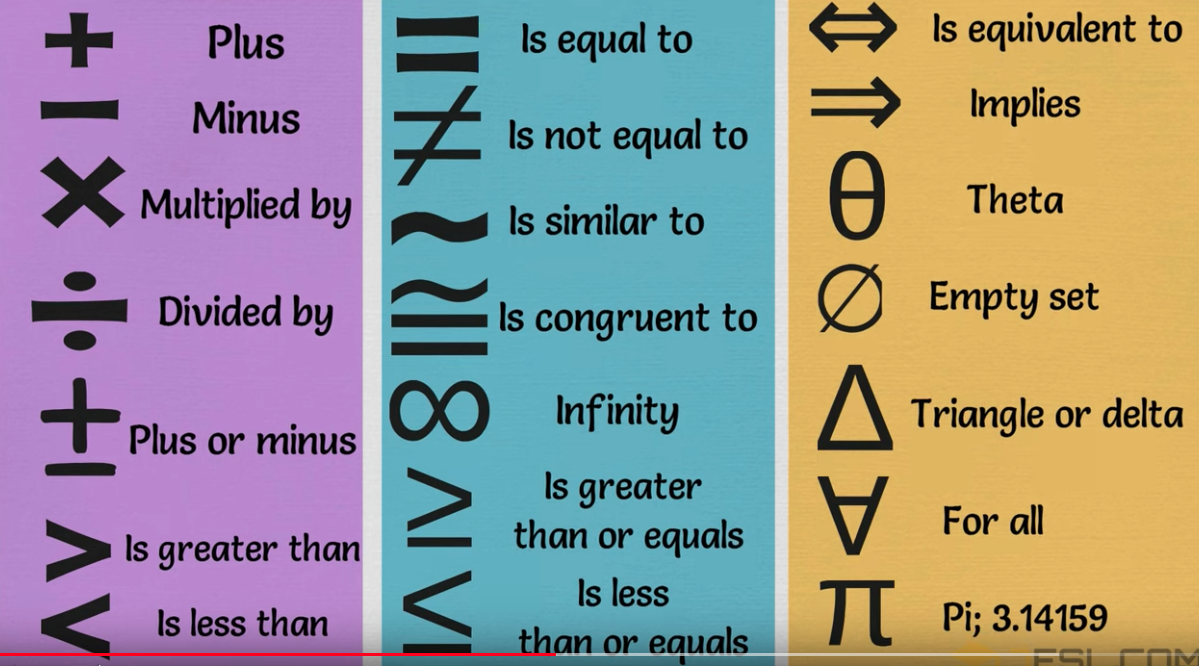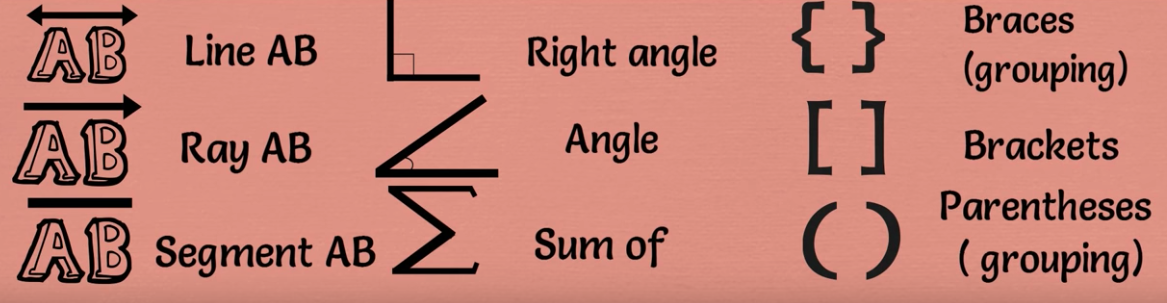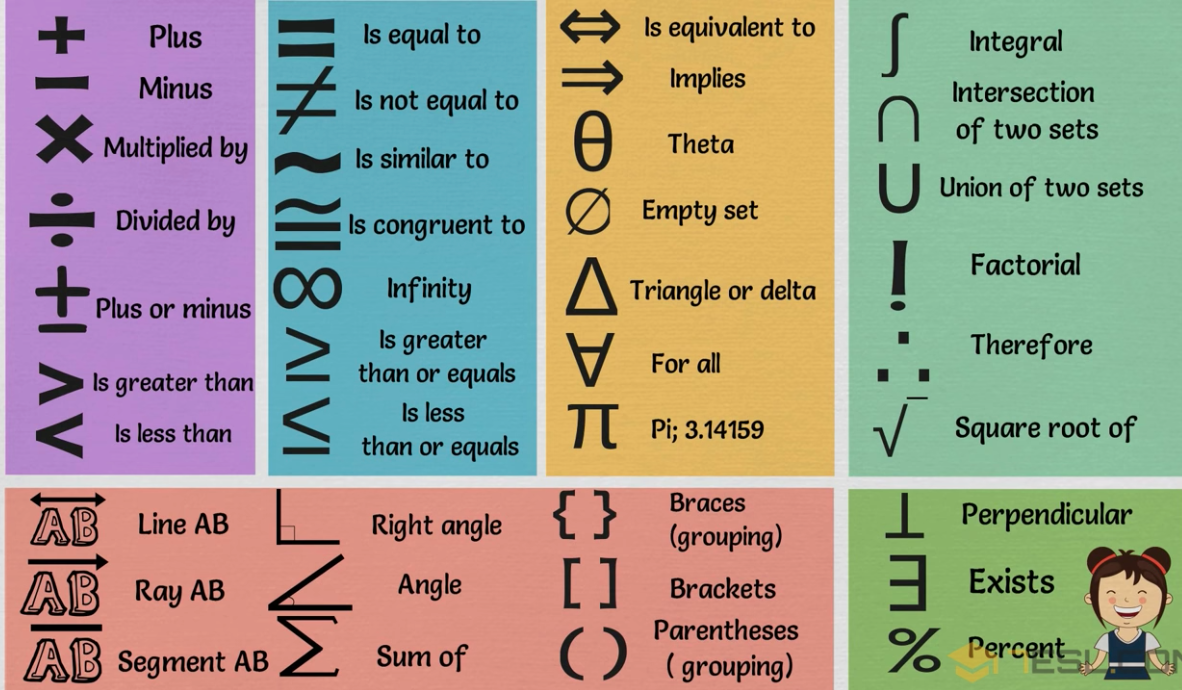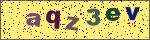# 符号英语

+ plus 加号；正号
- minus 减号；负号
± plus or minus 正负号
× is multiplied by 乘号
÷ is divided by 除号
＝ is equal to 等于号
≠ is not equal to 不等于号
≡ is equivalent to 全等于号
≌ is equal to or approximately equal to 等于或约等于号
≈ is approximately equal to 约等于号
＜ is less than 小于号
＞ is more than 大于号
≮ is not less than 不小于号
≯ is not more than 不大于号
≤ is less than or equal to 小于或等于号
≥ is more than or equal to 大于或等于号
% per cent 百分之...
‰ per mill 千分之...
∞ infinity 无限大号
∝ varies as 与...成比例
√ (square) root 平方根
∵ since; because 因为
∴ hence 所以
∷ equals, as (proportion) 等于，成比例
∠ angle 角
⌒ semicircle 半圆
⊙ circle 圆
○ circumference 圆周
π pi 圆周率
△ triangle 三角形
⊥ perpendicular to 垂直于
∪ union of 并，合集
∩ intersection of 交，通集
∫ the integral of ...的积分
∑ (sigma) summation of 总和
° degree 度
′ minute 分
″ second 秒
℃ Celsius system 摄氏度
{ open brace, open curly 左花括号
} close brace, close curly 右花括号
( open parenthesis, open paren 左圆括号
) close parenthesis, close paren 右圆括号
() brakets/ parentheses 括号
[ open bracket 左方括号
] close bracket 右方括号
[] square brackets 方括号
. period, dot 句号，点
| vertical bar, vertical virgule 竖线
& ampersand, and, reference, ref 和，引用
* asterisk, multiply, star, pointer 星号，乘号，星，指针
/ slash, divide, oblique 斜线，斜杠，除号
// slash-slash, comment 双斜线，注释符
# pound 井号
backslash, sometimes
escape 反斜线转义符，有时表示转义符或续行符
~ tilde 波浪符
. full stop 句号
, comma 逗号
: colon 冒号
; semicolon 分号
? question mark 问号
! exclamation mark (英式英语) exclamation point (美式英语)
' apostrophe 撇号
- hyphen 连字号
-- dash 破折号
... dots/ ellipsis 省略号
" single quotation marks 单引号
"" double quotation marks 双引号
‖ parallel 双线号
& ampersand = and
～ swung dash 代字号
§ section; division 分节号
→ arrow 箭号；参见号#### 发表评论